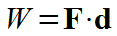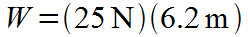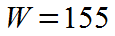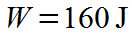### Calculate work given force and displacement.

#### Example question:

How much work is done by a force of 25 N that operates over a displacement of 6.2 m?

#### Given:

Force: F = 25 N

displacement: d = 6.2 mStarting formulaPlug in numbers with unitsMultiply: (25)(6.2) = 155Final result with significant figures and unit